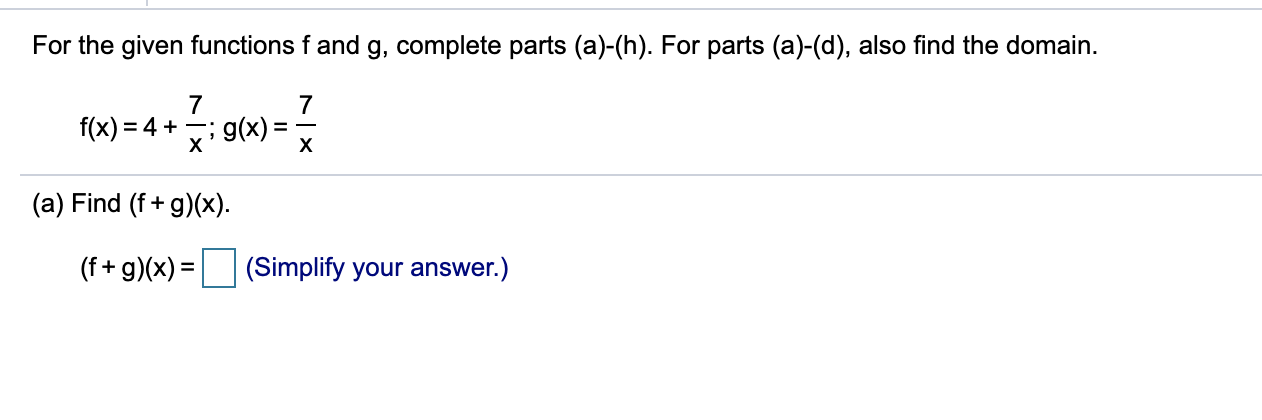# For the given functions f and g, complete parts (a)-(h). For parts (a)-(d), also find the domain.f(x) = 4 +х; g(x):х(a) Find (f + g)(x).(f + g)(x) =(Simplify your answer.)

Question
16 viewshelp_outlineImage TranscriptioncloseFor the given functions f and g, complete parts (a)-(h). For parts (a)-(d), also find the domain. f(x) = 4 + х ; g(x): х (a) Find (f + g)(x). (f + g)(x) = (Simplify your answer.) fullscreen
check_circle

Step 1

Given functions f and g are

Step 2

(a)

(f + g)(x) can be written as

Step 3

Therefore,

&nb...

### Want to see the full answer?

See Solution

#### Want to see this answer and more?

Solutions are written by subject experts who are available 24/7. Questions are typically answered within 1 hour.*

See Solution
*Response times may vary by subject and question.
Tagged in

### Other